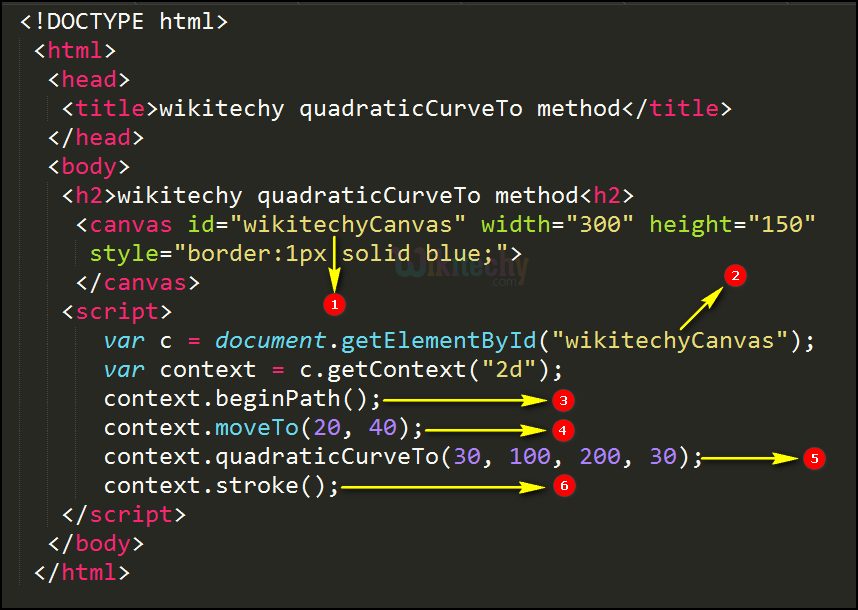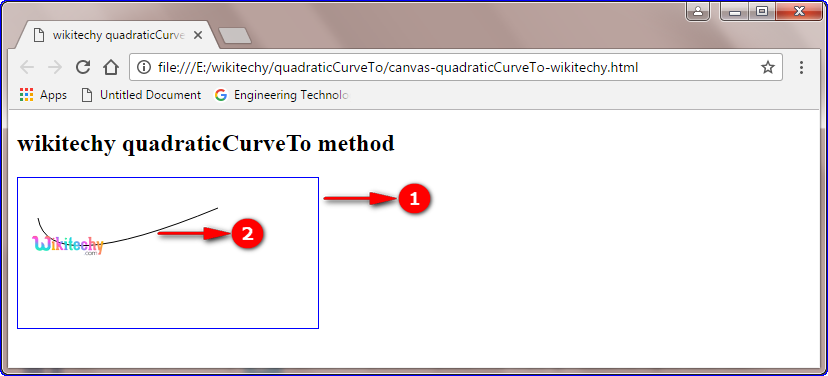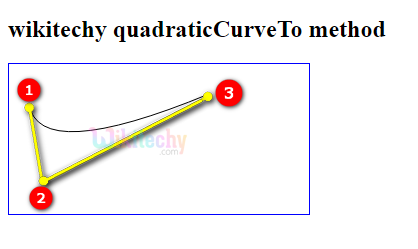# html tutorial - quadraticCurveTo() Method in HTML5 Canvas - html5 - html code - html form

• The quadraticCurveTo() is a method of HTML canvas.
• The quadraticCurveTo() method adds a point to the current path by using the specified control points that represent a quadratic parametric curve.

## Parameter values for quadraticCurveTo() Method in HTML5 Canvas:

Parameter Description
cpx The x-coordinate of the Bezier control point.
cpy The y-coordinate of the Bezier control point.
x The x-coordinate of the ending point.
y The y-coordinate of the ending point.

## Code Explanation for quadraticCurveTo() Method in HTML5 Canvas:1. “wikitechyCanvas” is declare id value of the HTML canvas.
2. The getElementById() method is used to get the element with the specific id (“wikitechyCanvas”).
3. The beginPath() method is used to begins a path.
4. The moveTo() method is used to moves the path to the specified point (20,40) in the canvas.
5. A quadratic parametric curve requires two points. The first point is a control point (30,100) that is used in the quadratic parametric calculation and the second point is the ending point (200,30) for the curve.
6. The stroke() method is used to draws a path by using moveTo() and quadraticCurveTo() methods.

## Output for quadraticCurveTo() Method in HTML5 Canvas:1. The canvas rectangle with blue color border.
2. The quadratic parametric curve is displayed on the canvas.1. Start point(20,40)
2. Control point(30,100)
3. End point(200,30)

## Browser Support for quadraticCurveTo() Method in HTML5 Canvas:Yes 9.0 Yes Yes Yes